Important Questions on Surface Area and Volume

Chapter 12 Class 10 Surface Areas and Volumes
Serial order wise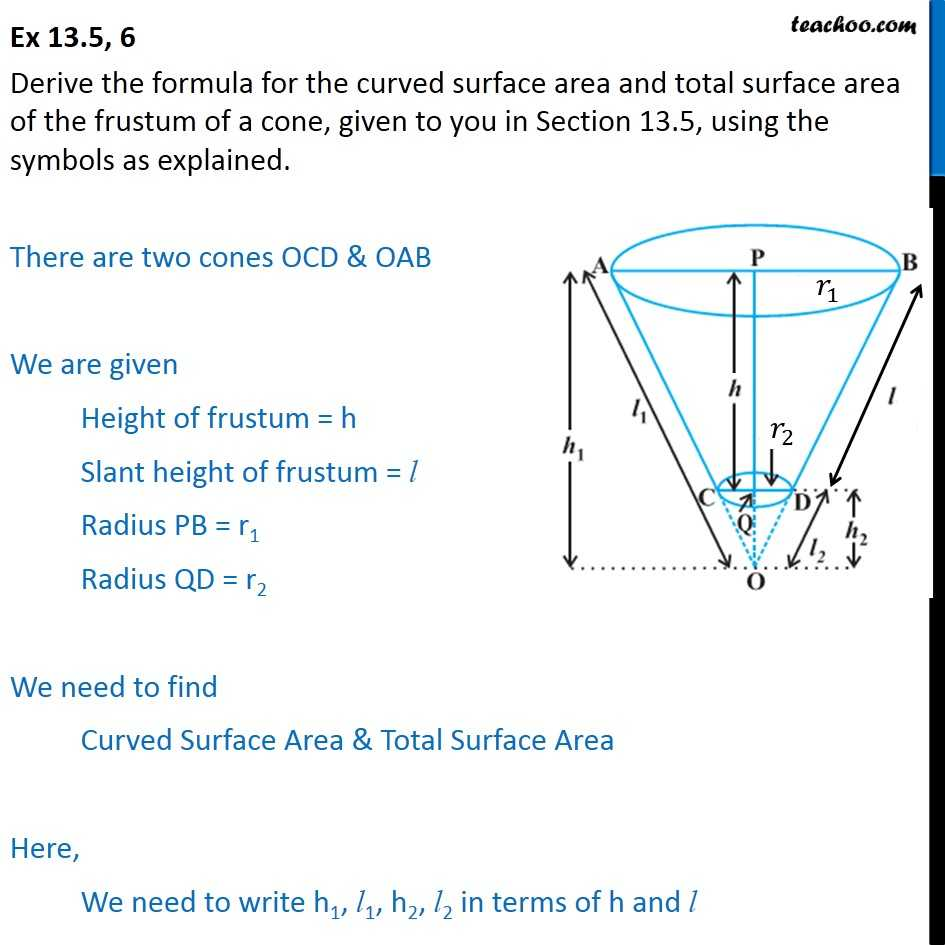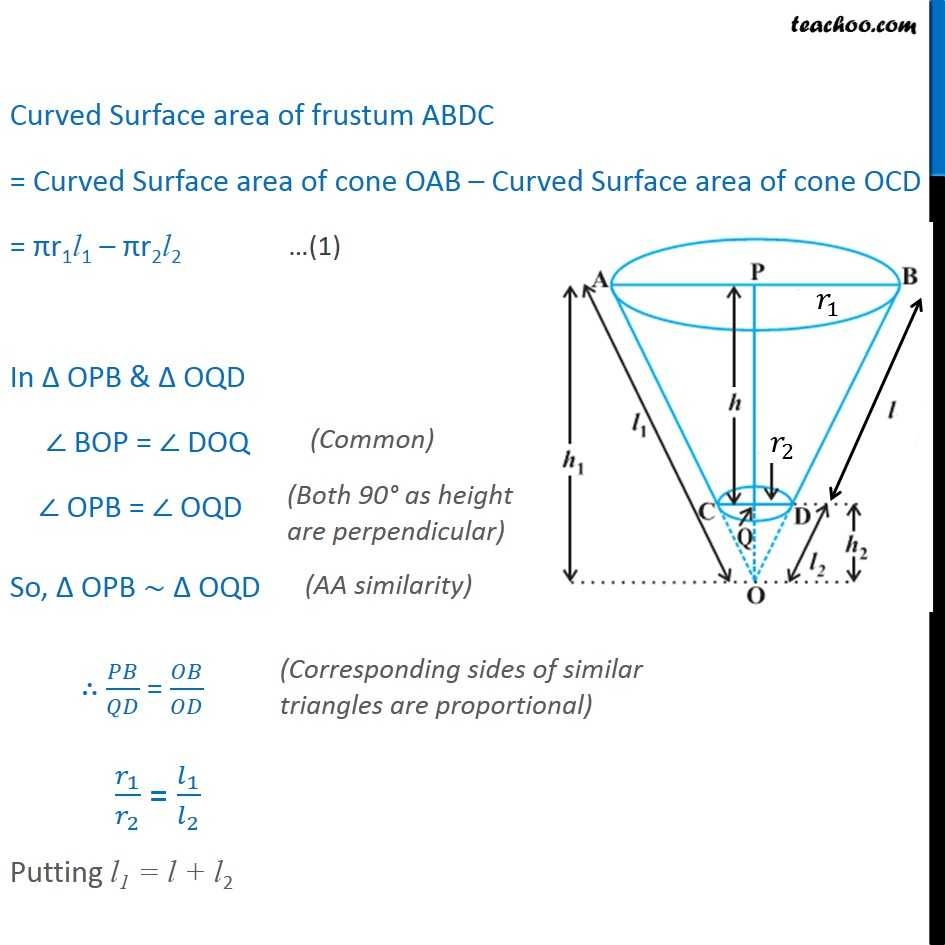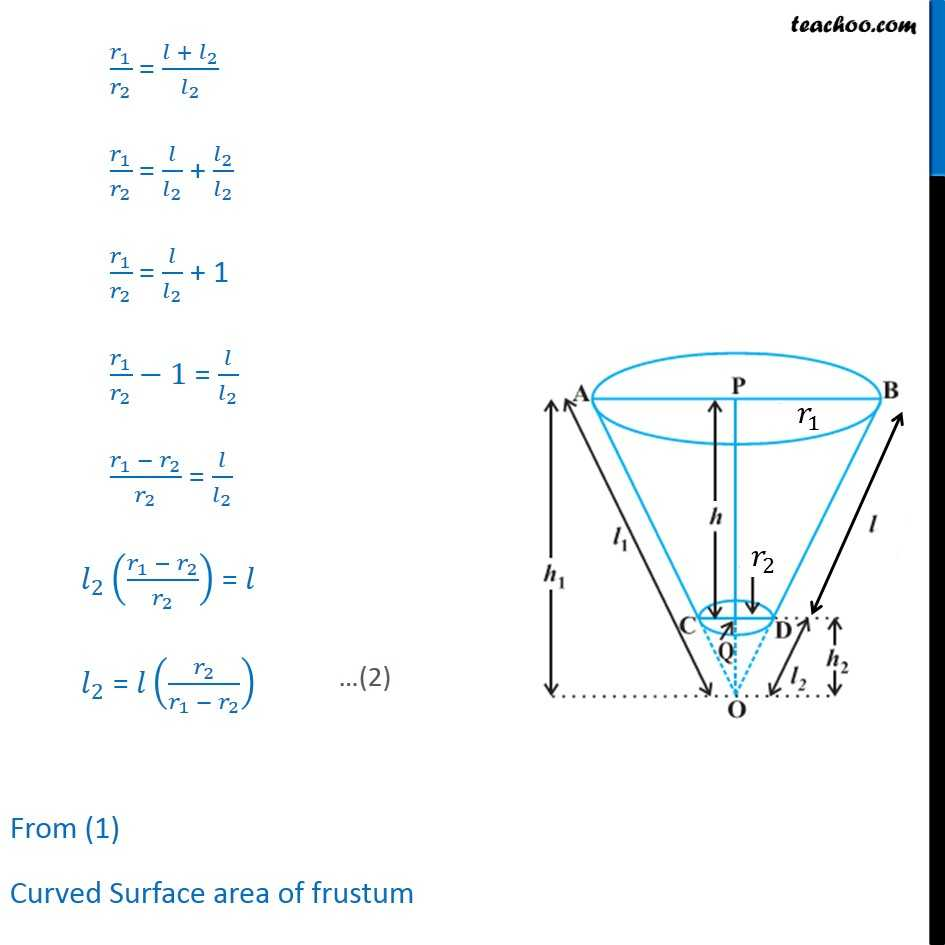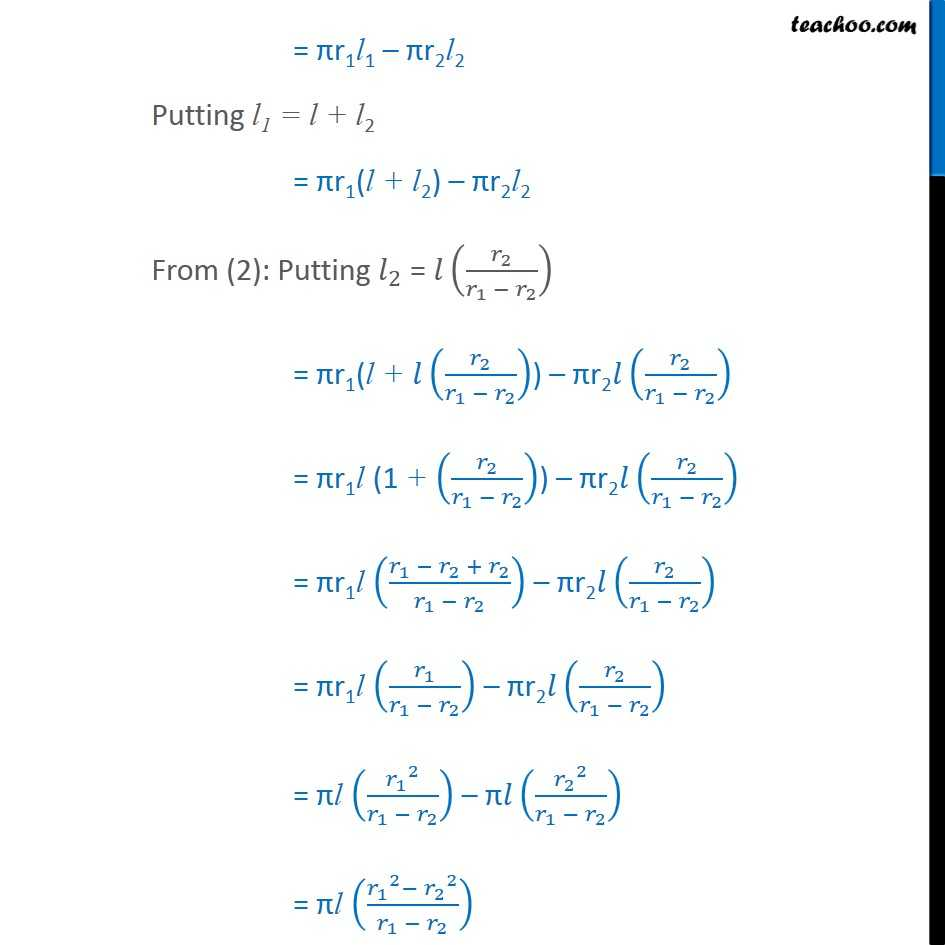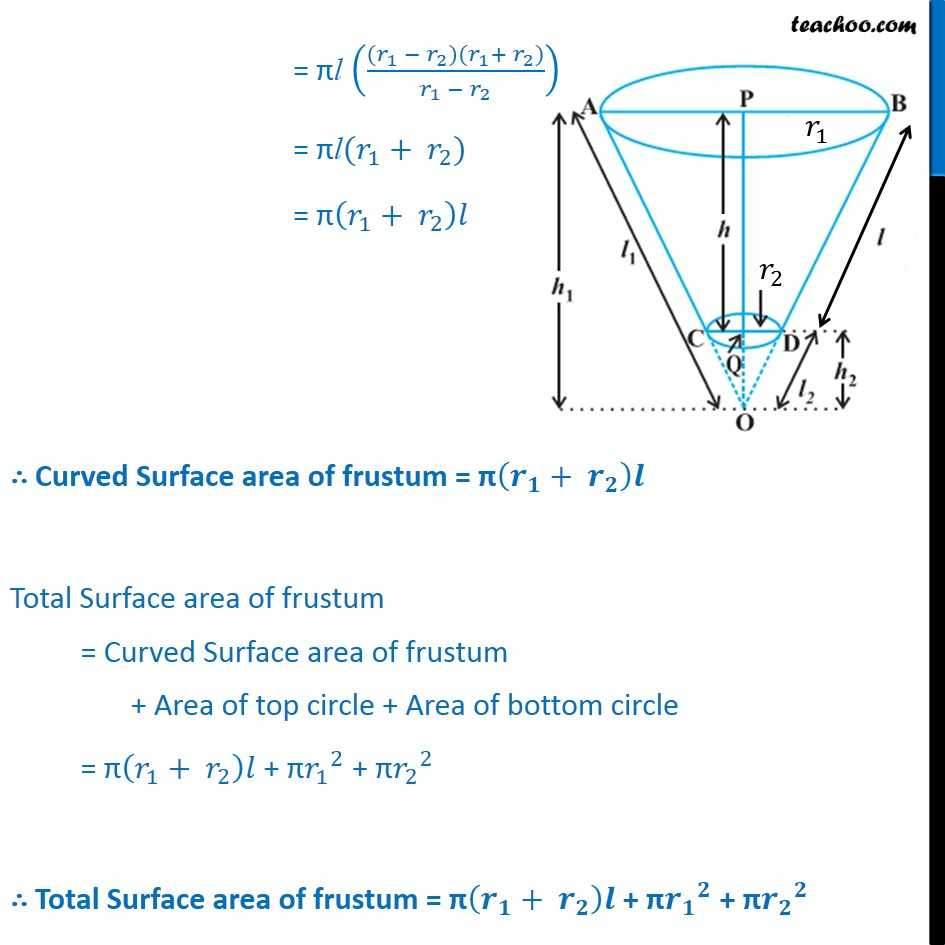Learn in your speed, with individual attention - Teachoo Maths 1-on-1 Class

### Transcript

Question 6 Derive the formula for the curved surface area and total surface area of the frustum of a cone, given to you in Section 13.5, using the symbols as explained. There are two cones OCD & OAB We are given Height of frustum = h Slant height of frustum = l Radius PB = r1 Radius QD = r2 We need to find Curved Surface Area & Total Surface Area Here, We need to write h1, l1, h2, l2 in terms of h and l Curved Surface area of frustum ABDC = Curved Surface area of cone OAB Curved Surface area of cone OCD = r1l1 r2l2 In OPB & OQD BOP = DOQ OPB = OQD So, OPB OQD / = / _1/ _2 = _1/ _2 Putting l1 = l + l2 _1/ _2 = ( + _2)/ _2 _1/ _2 = / _2 + _2/ _2 _1/ _2 = / _2 + 1 _1/ _2 1 = / _2 ( _1 _2)/ _2 = / _2 _2 (( _1 _2)/ _2 ) = _2 = ( _2/( _1 _2 )) From (1) Curved Surface area of frustum = r1l1 r2l2 Putting l1 = l + l2 = r1(l + l2) r2l2 From (2): Putting _2 = ( _2/( _1 _2 )) = r1(l + ( _2/( _1 _2 ))) r2 ( _2/( _1 _2 )) = r1l (1 + ( _2/( _1 _2 ))) r2 ( _2/( _1 _2 )) = r1l (( _1 _2 + _2)/( _1 _2 )) r2 ( _2/( _1 _2 )) = r1l ( _1/( _1 _2 )) r2 ( _2/( _1 _2 )) = l ( _1 ^2/( _1 _2 )) ( _2 ^2/( _1 _2 )) = l (( _1 ^2 _2 ^2)/( _1 _2 )) = l ((( _1 _2)( _1+ _2))/( _1 _2 )) = l( _1+ _2) = ( _1+ _2 ) Curved Surface area of frustum = ( _ + _ ) Total Surface area of frustum = Curved Surface area of frustum + Area of top circle + Area of bottom circle = ( _1+ _2 ) + _1 ^2 + _2 ^2 Total Surface area of frustum = ( _ + _ ) + _ ^ + _ ^